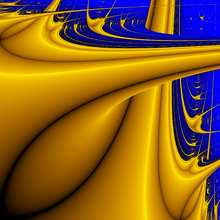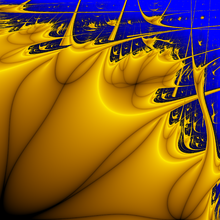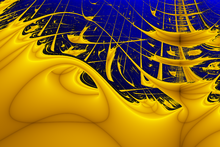# Lyapunov fractal

﻿
Lyapunov fractalStandard Lyapunov logistic fractal with iteration sequence AB, in the region [2, 4] × [2, 4].Generalized Lyapunov logistic fractal with iteration sequence AABAB, in the region [2, 4] × [2, 4].Generalized Lyapunov logistic fractal with iteration sequence BBBBBBAAAAAA, in the growth parameter region (A,B) in [3.4, 4.0] × [2.5, 3.4], known as Zircon Zity.

In mathematics, Lyapunov fractals (also known as Markus-Lyapunov fractals) are bifurcational fractals derived from an extension of the logistic map in which the degree of the growth of the population, r, periodically switches between two values A and B.

A Lyapunov fractal is constructed by mapping the regions of stability and chaotic behaviour (measured using the Lyapunov exponent λ) in the ab plane for a given periodic sequence of as and bs. In the images, yellow corresponds to λ < 0 (stability), and blue corresponds to λ > 0 (chaos).

## Properties

Lyapunov fractals are generally drawn for values of A and B in the interval [0,4]. For larger values, the interval [0,1] is no longer stable, and the sequence is likely to be attracted by infinity, although convergent cycles of finite values continue to exist for some parameters. For all iteration sequences, the diagonal a = b is always the same as for the standard one parameter logistic function.

The sequence is usually started at the value 0.5, which is a critical point of the iterative function. The other (even complex valued) critical points of the iterative function during one entire round are those that pass through the value 0.5 in the first round. A convergent cycle must attract at least one critical point[citation needed]; therefore all convergent cycles can be obtained by just shifting the iteration sequence, and keeping the starting value 0.5. In practice, shifting this sequence leads to changes in the fractal, as some branches get covered by others; notice for instance how the Lyapunov fractal for the iteration sequence AB is not perfectly symmetric with respect to a and b.

## Algorithm for generating Lyapunov fractals

An algorithm, for computing the fractal is summarized as follows.

1. Choose a string of As and Bs of any nontrivial length (e.g., AABAB).
2. Construct the sequence S formed by successive terms in the string, repeated as many times as necessary.
3. Choose a point$(a,b) \in [0,4] \times [0,4]$.
4. Define the function rn = a if Sn = A, and rn = b if Sn = B.
5. Let x0 = 0.5, and compute the iterates xn + 1 = rnxn(1 − xn).
6. Compute the Lyapunov exponent:$\lambda = \lim_{N \rightarrow \infty} {1 \over N} \sum_{n = 1}^N \log \left|{dx_{n+1} \over dx_n}\right| = \lim_{N \rightarrow \infty} {1 \over N} \sum_{n = 1}^N \log |r_n (1 - 2x_n)|$
In practice, λ is approximated by choosing a suitably large N.
7. Color the point (a,b) according to the value of λ obtained.
8. Repeat steps (3–7) for each point in the image plane.

Wikimedia Foundation. 2010.

### Look at other dictionaries:

• Lyapunov — may refer to:* Prokopy Lyapunov (? 1611), Russian statesman. * Zakhary Lyapunov (? after 1612), Russian statesman, Prokopy Lyapunov s brother. * Mikhail Lyapunov (1820 1868), Russian astronomer * Aleksandr Lyapunov (1857–1918), Russian… …   Wikipedia

• Fractal art — is created by calculating fractal objects and representing the calculation results as still images, animations, music, or other media.Fractal art is usually created indirectly with the assistance of a computer, iterating through three phases:… …   Wikipedia

• Fractal — A fractal is generally a rough or fragmented geometric shape that can be split into parts, each of which is (at least approximately) a reduced size copy of the whole, [cite book last = Mandelbrot first = B.B. title = The Fractal Geometry of… …   Wikipedia

• Fractal — Fractale Fractales On nomme fractale ou fractal (nom masculin moins usité), une courbe ou surface de forme irrégulière ou morcelée qui se crée en suivant des règles déterministes ou stochastiques impliquant une homothétie interne. Le terme… …   Wikipédia en Français

• Lyapunov exponent — In mathematics the Lyapunov exponent or Lyapunov characteristic exponent of a dynamical system is a quantity that characterizes the rate of separation of infinitesimally close trajectories. Quantitatively, two trajectories in phase space with… …   Wikipedia

• Fractal — Este artículo o sección sobre matemáticas necesita ser wikificado con un formato acorde a las convenciones de estilo. Por favor, edítalo para que las cumpla. Mientras tanto, no elimines este aviso puesto el 16 de octubre de 2010. También puedes… …   Wikipedia Español

• fractal — ● adj. ►MATH Se dit d objets mathématiques dont la création ou la forme ne trouve ses règles que dans l irrégularité ou la fragmentation, et des branches des maths qui étudient de tels objets. (© Larousse). Exemple: La vache qui rit est dans la… …   Dictionnaire d'informatique francophone

• Aleksandr Lyapunov — Infobox Scientist name = Aleksandr Mikhailovich Lyapunov caption = birth date = birth date|1857|6|6 birth place = Yaroslavl, Imperial Russia death date = death date and age|1918|11|3|1857|6|6 death place = residence = Russia citizenship =… …   Wikipedia

• List of fractal topics — This is a list of fractal topics, by Wikipedia page, See also list of dynamical systems and differential equations topics.*1/f noise *Apollonian gasket *Attractor *Box counting dimension *Cantor distribution *Cantor dust *Cantor function *Cantor… …   Wikipedia

• Fractale De Lyapunov — Pour les articles homonymes, voir Lyapunov. Fractale de Lyapunov avec la séquence AB …   Wikipédia en Français